## Handling structural breaks with logarithms

As we saw in other econometric blogs of M&S Research Hub, the use of logarithms constitutes a usual practice in econometrics, not only for the problems that can be derived from overusing them, but also it was mentioned the advantage to reduce the Heteroscedasticity -HT- (Nau, 2019) present in the series of a dataset, and some improvements that the monotonic transformation performs on the data as well.

In this article, we’re going to explore the utility of the logarithm transformation to reduce the presence of structural breaks in the time series context. First, we’ll review what’s a structural break, what are the implications of regressing data with structural breaks and finally, we’re going to perform a short empirical analysis with the Gross Domestic Product -GDP- of Colombia in Stata.

The structural break

We can define a structural break as a situation where a sudden, unexpected change occurs in a time series variable, or a sudden change in the relationship between two-time series (Casini & Perron, 2018). In this order of ideas, a structural change might look like this:

The basic idea is to identify abrupt changes in time series variables but we’re not restricting such identification to the domain of time, it can be detected also when we scatter X and Y variables that not necessarily consider the dependent variable as the time. We can distinguish different types of breaks in this context, according to Hansen (2012)  we can encounter breaks in 1) Mean, 2) Variance, 3) Relationships, and also we can face single breaks, multiple breaks, and continuous breaks.

Basic problems of the structural breaks

Without going into complex mathematical definitions of the structural breaks, we can establish some of the problems when our data has this situation. The first problem was identified by Andrews (1993) regarding to the parameter’s stability related to structural changes, in simple terms, in the presence of a break, the estimators of the least square regression tend to vary over time, which is of course something not desirable, the ideal situation is that the estimators would be time invariant to consolidate the Best Linear Unbiased Estimator -BLUE-.

The second problem of structural breaks (or changes) not taken in account during the regression analysis is the fact that the estimator turns to be inefficient since the estimated parameters are going to have a significant increase in the variance, so we’re not getting a statistical unbiased estimator and our exact inferences or forecasting analysis wouldn’t be according to reality.

A third problem might appear if the structural break influences the unit root identification process, this is not a wide explored topic but Tai-Leung Chong (2001) makes excellent appoints related to this. Any time series analysis should always consider the existence of unit roots in the variables, in order to provide further tools to handle a phenomenon, that includes the cointegration field and the forecasting techniques.

An empirical approximation

Suppose we want to model the tendency of the GDP of the Colombian economy, naturally this kind of analysis explicitly takes the GDP as the dependent variable, and the time as the independent variable, following the next form:

In this case, we know that the GDP expressed in Y is going to be a function of the time t. We can assume for a start that the function f(t) follows a linear approximation.

With this expression in (1), the gross domestic production would have an independent autonomous value independent of time defined in a, and we’ll get the slope coefficient in α which has the usual interpretation that by an increase of one-time unit, the GDP will have an increase of α.

The linear approximation sounds ideal to model the GDP against the changes over time, assuming that t has a periodicity of years, meaning that we have annual data (so we’re excluding stational phenomena); however, we shall always inspect the data with some graphics.

With Stata once we already tsset the database, we can watch the graphical behavior with the command “scatter y t”.

In sum, the linear approximation might not be a good idea with this behavior of the real GDP of the Colombian economy for the period of analysis (1950-2014). And it appears to be some structural changes judging by the tendency which changes the slope of the curve drastically around the year 2000.

If we regress the expression in (1), we’ll get the next results.

The linear explanation of the time (in years) related to the GDP is pretty good, around 93% of the independent variable given by the time, explains the GDP of the Colombian economy, and the parameter is significant with a level of 5%.

Now I want you to focus in two basic things, the variance of the model which is 1.7446e+09 and the confidence intervals, which positions the estimator between 7613.081 and 8743.697. Without having other values to compare these two things, we should just keep them in mind.

Now, we can proceed with a test to identify structural breaks in the regression we have just performed. So, we just type “estat sbsingle” in order to test for a structural break with an unknown date.

The interesting thing here is that the structural break test identifies one important change over the full sample period of 1950 to 2014, the whole sample test is called “supremum Wald test” and it is said to have less power than average or exponential tests. However, the test is useful in terms of simply identify structural terms which also tend to match with the graphical analysis. According to the test, we have a structural break in the year 2002, so it would be useful to graph the behavior before and after this year in order to conclude the possible changes.  We can do this with the command “scatter y t” and include some if conditions like it follows ahead.

` twoway (scatter Y t if t<=2002)(lfit  Y t if t<=2002)(scatter Y t if t>=2002)(lfit  Y t if t>=2002) `

We can observe that tendency is actually changing if we adjust the line for partial periods of time, given by t<2002 and t>2002, meaning that the slope change is a sign of structural break detected by the program. You can attend this issue including a dummy variable that would equal 0 in the time before 2002 and equal 1 after 2002. However, let’s graph now the logarithm transformation of GDP.  The mathematical model would be:

Applying natural logarithms, we got:

α now becomes the average growth rate per year of the GDP of the Colombian economy, to implement this transformation use the command “gen ln_y=ln(Y)” and the graphical behavior would look like this:

``` gen ln_Y=ln(Y)
scatter ln_Y t```

The power of the monotonic transformation is now visible, there’s a straight line among the variable which can be fitted using a linear regression, in fact, let’s regress the expression in Stata.

Remember that I told you to keep in mind the variance and the confidence intervals of the first regression? well now we can compare it since we got two models, the variance of the last regression is 0.0067 and the intervals are indeed close to the coefficient (around 0.002 of difference between the upper and lower interval for the parameter). So, this model fits even greatly than the first.

If we perform again the “estat sbsingle” test again, it’s highly likely that another structural break might appear. But we should not worry a lot if this happens, because we rely on the graphical analysis to proceed with the inferences, in other words, we shall be parsimonious with our models, with little, explain the most.

The main conclusion of this article is that the logarithms used with its property of monotonic transformation constitutes a quick, powerful tool that can help us to reduce (or even delete) the influences of structural breaks in our regression analysis. Structural changes are also, for example, signs of exogenous transformation of the economy, as a mention to apply this idea for the Colombian economy, we see it’s growing speed changing from 2002 until the recent years, but we need to consider that in 2002, Colombia faced a government change which was focused on the implementation of public policies related to eliminating terrorist groups, which probably had an impact related to the investment process in the economy and might explain the growth since then.

### Bibliography

Andrews, D. W. (1993). Tests for Parameter Instability and Structural Change With Unknown Change Point. Journal of the Econometric Society Vol. 61, No. 4 (Jul., 1993), 821-856.

Casini, A., & Perron, P. (2018). Structural Breaks in Time Series. Retrieved from Economics Department, Boston University: https://arxiv.org/pdf/1805.03807.pdf

Nau, R. (2019). The logarithm transformation. Retrieved from Data concepts The logarithm transformation: https://people.duke.edu/~rnau/411log.htm

Shresta, M., & Bhatta, G. (2018). Selecting appropriate methodological framework for time series data analysis. Retrieved from The Journal of Finance and Data Science: https://www.sciencedirect.com/science/article/pii/S2405918817300405

Tai-Leung Chong, T. (2001). Structural Change In Ar(1) Models. Retrieved from Econometric Theory,17. Printed in the United States of America: 87–155

## Robust Modeling of Policy Changes: Difference in Difference (DiD)

The difference in Difference (DiD) is a popular method in empirical economics and has important applications in other social sciences as well. DID is a quasi-experimental design that uses panel data to estimate the effects of specific intervention or treatment (such as policy changes, new laws, social program implementation) on outcomes over time and between two population groups, those that are affected by this policy and those that are not. DiD, in general, is an appealing choice for researchers who want to design a research methodology based on controlling for confounding variables.

Applications of DiD are quite diverse, amongst are

• Impact evaluation (public policy analysis)
• Measuring the variations overtime (Time Series) and over individuals (Cross-Sectional Data)
• Focusing on the establishment of effects on a dependent variable derived from the interaction of exogenous variables given a treatment.
• Variants of the DiD method can account to deal with auto-selection bias and endogeneity problems.
• Comparing the differences between observed outcomes from partial and non-randomized samples in groups.

Although this method is highly important, few learning resources are available to instruct researchers and scientists how to properly implement and design it. There may be some resources that discuss the theoretical foundations of this method while listing a few examples of its applications. However a fully-fledged learning material for DiD that covers both theory, and guides researchers to implement this method on statistical software using real and simulated data applications are rather scarce.

At M&S Research Hub we recently launched a video library wherein our team of academic experts record offline training videos for advanced econometrics methods. This material is designed to fit researchers at different proficiency levels. They cover both theoretical and mathematical basics of the target models and their detailed application using statistical software, leaving the researcher in no further need to search for other learning resources.

A complete DiD course that takes around 158 minutes and is recorded over 7 videos are available in the library for everyone who wants to master the DID method.

## Taking Logarithms of Growth Rates and Log-based Data.

A usual practice while we’re handling economic data, is the use of logarithms, the main idea behind using them is to reduce the Heteroscedasticity -HT- of the data (Nau, 2019). Thus reducing HT, implies reducing the variance of the data. Several times, different authors implement some kind of double logarithm transformation, which is defined as taking logarithms of the data which is already in logarithms and growth rates (via differencing logarithms).

The objective of this article is to present the implications of this procedures, first by analyzing what does do the logarithm to a variable, then observing what possible inferences can be done when logarithms are applied to growth rates.

There are a series of properties about the logarithms that should be considered first, we’re not reviewing them here, however the reader can check them in the following the citation (Monterey Institute, s.f). Now let’s consider a bivariate equation:

The coefficient B represents the marginal effect of a change of one unit in X over Y. So, interpreting the estimation with ordinary least squares estimator gives the following analysis: When x increases in one unit, the result is an increase of B in y. It’s a lineal equation where the marginal effect is given by:

When we introduce logarithms to the equation of (1) by modifying the functional form, the estimation turns to be non-linear. However, let’s first review what logarithms might do to the x variable. Suppose x is a time variable which follows an upward tendency, highly heteroscedastic as the next graph shows.

We can graphically appreciate that variable x has a positive trend, and also that has deviations over his mean over time. A way to reduce the HT present in the series is to make a logarithm transformation. Using natural logarithms, the behavior is shown in the next graph.

The units have changed drastically, and we can define that logarithm of x is around 2 and 5. Whereas before we had it from 10 to 120 (the range has been reduced). The reason, the natural logarithm reduces HT because the logarithms are defined as a monotonic transformation (Sikstar, s.f.). When we use this kind of transformation in econometrics like the following regression equation:

The coefficient B is no longer the marginal effect, to interpret it we need to divide it by 100 (Rodríguez Revilla, 2014). Therefore, the result should be read as: an increase of one unit in x produces a change of B/100 in y.

If we use a double-log model, equation can be written as:

In this case, the elasticity is simply B which is interpreted in percentage. Example, if B=0.8. By an increase of 1% in x, the result would be an increase of 0.8% in y.

On the other hand, if we use log-linear model, equation can be written as:

In this case, B must be multiplied by 100 and it can be interpreted as a growth rate in average per increases of a unit of x. If x=t meaning years, then B is the average growth per year of y.

The logarithms also are used to calculate growth rates. Since we can say that:

The meaning of equation (5) is that growth rates of a variable (left hand of the equation) are approximately equal to the difference of logarithms. Returning with this idea over our x variable in the last graphic, we can see that the growth rate between both calculations are similars.

It’s appreciably the influence of the monotonic transformation; the growth rate formula has more upper (positive) spikes than the difference of logarithms does. And inversely the lower spikes are from the difference of logarithms.  Yet, both are approximately growth rates which indicate the change over time of our x variable.

For example, let’s place on the above graphic when is the 10th year.  The difference in logarithms indicates that the growth rate is -0.38% while the growth rate formula indicates a -0.41% of the growth-related between year 9th and now.  Approximately it’s 0.4% of negative growth between these years.

When we use logarithms in those kinds of transformations we’ll get mathematically speaking, something like this:

Some authors just do it freely to normalize the data (in other words reducing the HT), but Would be the interpretation remain the same? What are the consequences of doing this? It’s something good or bad?

As a usual answer, it depends. What would happen if, for example, we consider the years 9 and 10 again of our original x variable, we can appreciate that the change it’s negative thus the growth rate it’s negative. Usually, we cannot estimate a logarithm when the value is negative.

With this exercise, we can see that the first consequence of overusing logarithms (in differenced logarithms and general growth rates) is that if we got negative values, the calculus becomes undefined, so missing data will appear. If we graph the results of such thing, we’ll have something like this:

At this point, the graphic takes the undefined values (result of the logarithm of negative values) as 0 in the case of Excel, other software might not even place a point.  We got negative values of a growth rate (as expected), but what we got now is a meaningless set of data. And this is bad because we’re deleting valuable information from other timepoints.

Let’s forget for now the x variable we’ve been working with.  And now let’s assume we got a square function.

The logarithm of this variable since its exponential would be:

and if we apply another log transformation, then we’ll have:

However, consider that if z=0, the first log would be undefined, and thus, we cannot calculate the second. We can appreciate this in some calculations as the following table shows.

The logarithm of 0 is undefined, the double logarithm of that would be undefined too. When z=1 the natural logarithm is 0, and the second transformation is also undefined. Here we can detect another problem when some authors, in order to normalize the data, apply logarithms indiscriminately. The result would be potential missing data problem due to the monotonic transformation when values of the data are zero.

Finally, if we got a range of data between 0 and 1, the logarithm transformation will induce the calculus to a negative value. Therefore, the second logarithm transformation it’s pointless since all the data in this range is now undefined.

The conclusions of this article are that when we use logarithms in growth rates, one thing surely can happen: 1) If we got potential negative values in the original growth rate, and then apply logarithms on those, the value becomes undefined, thus missing data that will occur. And the interpretation becomes harder. Now if we apply some double transformation of log values, the zero and the negative values in the data will become undefined, thus missing data problem will appear again. Econometricians should take this in considerations since it’s often a question that arises during researches, and in order to do right inferences, analyzing the original data before applying logarithms should be a step before doing any econometric procedure.

### Bibliography

Monterey Institute. (s.f). Properties of Logarithmic Functions. Obtained from: http://www.montereyinstitute.org/courses/DevelopmentalMath/TEXTGROUP-1-19_RESOURCE/U18_L2_T2_text_final.html

Nau, R. (2019). The logarithm transformation. Obtenido de Data concepts The logarithm transformation. Obtained from: https://people.duke.edu/~rnau/411log.htm

Rodríguez Revilla, R. (2014). Econometria I y II. Bogotá. : Universidad Los Libertadores.

Sikstar, J. (s.f.). Monotonically Increasing and Decreasing Functions: an Algebraic Approach. Obtained from: https://opencurriculum.org/5512/monotonically-increasing-and-decreasing-functions-an-algebraic-approach/

## The Development Impact of Foreign Aid: Story Re-told

There has been an intense debate in the literature over the reasons behind the loose developmental effects of foreign aid. Away from the straightforward reason that majority of aid flows follow political rather than development objectives (Kanbur et al., 1999; Dreher, et al. 2009). Further, several reasons have been introduced in the literature see for example (Radelet, 2008; Bräutigam and Knack, 2004, and others). However, these reasons can be condensed into two core causes, a) Lack of clear development agenda by the recipient countries which result in misallocation of aid and establishment of various simultaneous individual projects that even if successfully completed will have limited impact on the development of the target sectors; and b) The mismatch between donors, recipients, and target beneficiaries’ priorities and needs that create an aid system that is incapable and inadequate of achieving the entailed goals.

In response to common pitfalls of the aid system, a new setting for aid disbursements based on sector-wide approaches instead of individual projects was introduced by Kamel, et al. (1998) and was later modified by (Kanbur, et al. 1999) and evolved into a system entitled the ‘common pool approach’. Our following policy suggestions will build on these, however, we bring in some modifications for the system implementation and evaluation.

The basic rationale behind the common pool approach is that a pool of donors—instead of one— allocates unconditional funds to a recipient country’s nationally representative reform plan and its implementation strategy, instead of individual projects. Such a system would increase the recipient country’s sense of ownership and commitment while enhancing the achievability of developmental and reform goals relative to individual uncoordinated projects approach. The major drawback is the minimisation of aid received because many donors – besides political lobbies and private sector firms – might not agree to fund national plans instead of individual selective projects. In addition, donors’ ability to pursue their own interests, conditions, and opinions will dwindle. Anyhow, detailed discussion for the common pool system and sector-wide approach is found in formerly cited articles. Subsequently, we introduce a new system which combines both approaches, sector-wide and common pool, whilst including our personal reflections that will hopefully alleviate the expected drawbacks of these approaches.

Figure A1 provides a basic graphical representation of the new blended system. The graph is elaborated in the following points,

1. The recipient country starts to move in the direction of prohibiting all forms of aid transferred to individual uncoordinated projects, but rather allows only aid channeled towards sectoral reform plans.
• The governmental authority with the cooperation of civil society, the private sector, policymakers, and citizens, formulate a reform plan for the target sector.
• A series of roundtable meetings are held in the recipient country capital that involves potential donors (single and multilateral), international experts, and other national parties in order to receive feedback on the preliminary proposal (sponsoring the meetings in the recipient country would ease national parties’ involvement and cooperation, which reflects in a higher sense of belonging and ownership).
• A final neat version of the proposal is then reformulated along with its implementation strategy that involves foreign and domestic shares. For instance, technical and human resources in the implementation strategy are distributed as 70% domestic and 30% by the donor’s side. One major drawback of the common pool approach is the lack of donor involvement in the implementation, which in essence is a good thing to increase the sense of ownership by the recipient. However, this is reflected in lower lobbying by the private sector and political parties in the donor country to step forward for similar approaches. We, therefore, propose a cooperative share of interests, however still managed and authorised by the domestic country and in the framework of the domestic strategy.
• The donor authority in this phase lies in accepting or rejecting the plan and the amount of fund provided, based on credibility and achievability of the plan.
• Donors together with the recipient responsible authority would agree on a set of quantifiable and measurable assessment measures that are monitored and reported by the authority itself, though donors are also allowed to intervene in the monitoring and evaluation of these measures. This is an incentive for the authority and other parties involved in the strategy to abide by the rules and the plan. Also, in the case of system corruption, which is the likely case in the majority of developing and poor countries, it is well known that foreign assessment might intervene anytime to inspect and evaluate. In addition, donors will be more relieved and secure when they have a hand in the evaluation process, unlike the common pool approach which prohibits any form of foreign intervention in the process unless requested by the recipient.
• Finally, a renewable annual funding plan is offered based on the realisation of these measures; failure to abide by the authority results in a violation of the contract. By doing this we eliminate any chances of aid misallocation, corruptive activities, and other illegal traits because the recipient knows for sure that failure will hinder any future possibilities of funding for other reform plans. Moreover, the ex-ante participation of civil society and citizens makes the government accountable to the public, which also affects their political popularity.

Eventually, let me conclude with this phrase from Kanbur, et al. (1999) “The possibility of the decline in aid will require a substantial amount of confidence on the part of recipients who adopt the approach. It requires a government with the willpower to say to donors: ‘Here is my program in this sector: if you wish to help me implement it, you are most welcome. If you wish to do something different, I regret that you are not welcome in this sector in this country.” The foremost outcome of the proposed blended system, common pool, and sector-wide approaches, is filtration of aid received by the recipient, by adopting these approaches, will be able to locate donors that endeavor no hidden, political, or ideological agendas but only support the recipient country’s development efforts.

References:

Hassan, Sherif (2020). Revisiting the Development Impact of Sectorally Disaggregated Foreign Aid. Poverty and Public Policy (in press).

## Can cointegration analysis solve spurious regression problem?

The efforts to avoid the existence of spurious regression has led to the development of modern time series analysis (see How Modern Time Series Analysis Emerged? ). The core objective of unit root and cointegration procedures is to differentiate between genuine and spurious regression. However, despite the huge literature, the unit root and cointegration analysis are unable to solve spurious regression problem. The reason lies mainly in the misunderstanding of the term spurious regression.

Spurious correlation/spurious correlation occur when a pair of variable having no (weak) causal connection appears to have significant (strong) correlation/regression. In these meanings the term spurious correlation/spurious has the same history as the term regression itself. The correlation and regression analysis were invented by Sir Francis Galton in around 1888 and in 1897, Karl Pearson wrote a paper with the following title, ‘Mathematical Contributions to the Theory of Evolution: On a Form of Spurious Correlation Which May Arise When Indices Are Used in the Measurement of Organs’ (Pearson, 1897).

This title indicates number of important things about the term spurious correlation: (a) the term spurious correlation was known as early as 1897, that is, in less than 10 years after the invention of correlation analysis (ii) there were more than one types of spurious correlation known to the scientists of that time, therefore, the author used the phrase ‘On a Farm of Spurious Regression’, (c) the spurious correlation was observed in measurement of organs, a cross-sectional data (d) the reason of spurious correlation was use of indices, not the non-stationarity.

One can find in classical econometric literature that that many kinds of spurious correlations were known to experts in first two decades of twentieth century. These kinds of spurious correlations include spurious correlation due to use of indices (Pearson, 1897), spurious correlation due to variations in magnitude of population (Yule, 1910), spurious correlation due to mixing of heterogeneous records (Brown et al, 1914), etc. The most important reason, as the econometricians of that time understand, was the missing third variable (Yule, 1926).

Granger and Newbold (1974) performed a simulation study in which they generated two independent random walk time series x(t)=x(t-1)+e(t) and y(t)=y(t-1)+u(t) . The two series are non-stationary and the correlation of error terms in the two series is zero so that the two series are totally independent of each other. The two variables don’t have any common missing factor to which the movement of the two series can be attributed. Now the regression of the type y(t)=a+bx(t)+e(t) should give insignificant regression coefficient, but the simulation showed very high probability of getting significant coefficient. Therefore, Granger and Newbold concluded that spurious regression occurs due to non-stationarity.

Three points are worth considering regarding the study of Granger and Newbold. First, the above cited literature clearly indicates that the spurious correlation does exist in cross-sectional data and the Granger-Newbold experiment is not capable to explain cross-sectional spurious correlation. Second, the existing understanding of the spurious correlation was that it happens due to missing variables and the experiment adds another reason for the phenomenon which cannot deny the existing understanding. Third, the experiment shows that non-stationarity is one of the reasons of spurious regression. It does not prove that non-stationarity  is ‘the only’ reason of spurious regression.

However, unfortunately, the econometric literature that emerged after Granger and Newbold, adapted the misconception. Now, many textbooks discuss the spurious regression only in the context of non-stationarity, which leads to the misconception that the spurious regression is a non-stationarity related phenomenon. Similarly, the discussion of missing variable as a reason of spurious regression is usually not present in the recent textbooks and other academic literature.

To show that spurious regression is not necessarily a time series phenomenon, consider the following example:

A researcher is interested in knowing the relationship between shoe size and mathematical ability level of school students. He goes to a high school and takes a random sample of the students present in the school. He takes readings on shoe size and ability to solve the mathematical problems of the selected students. He finds that there is very high correlation between two variables. Would this be sufficient to argue that the admission policy of the school should be based on the measurement of shoe size? Otherwise, what accounts for this high correlation?

If sample is selected from a high school having kids in different classes, the same observation is almost sure to occur. The pupil in higher classes have larger shoe size and have higher mathematical skills, whereas student in lower classes have lower mathematical skills. Therefore, high correlation is expected. However, if we take data of only one class, say grade III, we will not see such high correlation. Since theoretically, there should be no correlation between shoe size and mathematical skills, this apparently high correlation may be regarded as spurious correlation/regression. The reason for this spurious correlation is mixing of missing age factor which drives both shoe size and mathematical skills.

Since this is not a time series data, there is no question of the existence of non-stationarity, but the spurious correlation exists. This shows that spurious correlation is no necessarily a time series phenomenon. The unit root and cointegration would be just incapable to solve this problem.

Similarly, it can be shown that the unit root and cointegration analysis can fail to work even with time series data, and this will be discussed in our next blog

## The impact of functional form over the normality assumption in the residuals

A discussed solution in order to accomplish the normality assumption in regression models relates to the correct specification of a Data Generating Process (Rodríguez Revilla, 2014), the objective here is to demonstrate how functional form might influence the distribution of the residuals in a regression model using ordinary least squares technique.

Let’s start with a Monte Carlo exercise using the theory of Mincer (1974) in which we have a Data Generating Process -DGP- of the income for a cross-sectional study of a population of a city.

With

The DGP expressed in (1) is the correct specification of income for the population of our city. Where y is the income in monetary units, schooling is the years of school of the individual, exp is the number of years of experience in the current job. Finally, we got the square of the experience which reflects by the negative sign, the decreasing returns of the variable over the income.

Let’s say we want to study the income in our city, so one might use a simple approximation model for the regression equation. In this case, we know by some logic that schooling and experience are related to the income, so we propose to use the next model in (2) to study the phenomena.

Regressing this model with our Monte Carlo exercise with the specification in (2) we got the next results, considering a sample size of 1000 individuals.

We can see that coefficients of the experience and the constant term are not so close to the DGP process, and that the estimator of schooling years on the other hand it’s approximately accurate. All variables are relevant at a 5% significance level and R^2 is pretty high.

We want to make sure if we got the right variables, so we use Ramsey RESET test to check if we got a problem of omitted variables. Let’s predict first the residuals with predict u, res of the above regression and then perform the test of omitted variables (using Ramsey omitted variable test with estat ovtest):

Ramsey test indicates no omitted variables at a 5% level of significance, so we have now an idea that we’re using the right variables. Let’s check out now, the normality assumption with a graphic distribution of the predicted residuals, in Stata we use the command histogram u, norm

Graphically the result shows that the behavior of the residuals is non-normal. In order to confirm this, we perform a formal test with sktest u and we’ll see the following results.

The test of normality of the residuals is not good. Meaning that with a 5% of the significance level of the error, the predicted residuals have a non-normal distribution. This invalidates the results of the t statistics in the coefficients in the regression of equation (2).

We should get back to our functional form in the regression model in (2), and now we should consider that experience might have some decreasing or increasing returns over the income. So, we adapt our specification including the square term of the experience to capture the marginal effect of the variable:

Now in order to regress this model in Stata, we need to generate the squared term of the experience. To do this we type gen exp_sq=experience*experience where experience is our variable.

We have now our squared variable of experience which we include the regression command as the following image presents.

We can see that coefficients are pretty accurate to the DGP of (1), which is because the specification is closer to the real relationship of the variables in our simulated exercise. The negative sign in the squared term indicates a decreasing return of experience over the income, and the marginal effect is given by:

Let’s predict our residuals of our new regression model with predict u2,res and let’s check the distribution of the residuals using histogram u2, norm

Residuals by graphic inspection presents a normal distribution, we confirm this with the formal test of normality with the command sktest u2

According to the last result we cannot reject the null hypothesis of a normal distribution in the predicted residuals of our second regression model, so we accept that residuals of our last estimates have a normal distribution with a 5% significance level.

The conclusion of this exercise is that even if we have the right variables for a regression model, just like we considered in equation (2), if the specification functional form isn’t correct then the behavior of the residuals will be not be normally distributed.

A correction in the specification form of the regression model can be considered as a solution for non-normality problems, since the interactions of the variables can be modeled better. However in real estimations, finding the right functional form is frequently harder and it’s attached to problems of the data, non-linear relationships, external shocks and atypical observations, but it worth the try to inspect the data in order to find what could be the proper functional form of the variables in order to establish a good regression model which come as accurate as possible to the data generating process.

##### References

Mincer, J. (1974). Education, Experience and the Distribution of Earnings and of employment. New York: National bureau of Economic Research (for the Carnegie Comission).

Rodríguez Revilla, R. (2014). Econometria I y II. Bogotá. Colombia : Universidad Los Libertadores.

StataCorp (2017) Stata Statistical Software: Release 15. College Station, TX: StataCorp LLC. Avaliable in: https://www.stata.com/products/

## How Modern Time Series Analysis Emerged?

The regression analysis which is basic tool of econometrics was invented in 1880s by Francis Galton, a cousin of Charles Darwin who is famous for his theory of evolution. Like Darwin, Galton was a biologist interested in the laws of heredity, and he intended to use the regression for the laws of heredity. He used the regression for analysis of cross-sectional data of the heights of fathers and sons. The regression analysis was than adapted and developed by the economists for analysis of economic data without any discrimination of time series and cross-sectional data.

Soon it was discovered that the application of regression analysis to the time series data could produced misleading results. In particular, regression analysis applied to time series data sometimes shows the two series to be highly correlated, when in fact there is no sensible economic relationship between the variables. This phenomenon was termed as ‘spurious regression’.

Yule (1926) wrote a detailed commentary on the spurious regression in time series. He gave number of examples in which two independent time series appear to be highly correlated. One of his examples was the relationship in marriages in Church of England and mortality rate. Obviously, the two variables don’t have any causal connection, but Yule fond 95% correlation between two variables. Yule thought that this phenomenon is because of some missing variable and could be avoided by taking into account all relevant variables. He further assumed that spurious regression would disappear if longer time series are available. This means by increasing the time series length, the chances of spurious regression will gradually diminish. In coming half century, the missing variable was thought as the main reason for the spurious correlation in time series.

In 1974, Granger and Newbold observed that in case of non-stationary time series, the spurious regression may exist even if there is no missing variable. They further found that the probability of spurious regression increases by increasing the time series length, contrary to the perception of Yule who had thought that probability of spurious regression will decrease with the increase in time series length. A few years later in 1982, Nelson and Plossor analyzed a set of time series of the United States and found that most of these series are non-stationary. Many other studies supported the finding of Nelson and Plossor creating a doubt about stationarity of time series.

If one combines the finding of Granger and Newbold with that of Nelson and Plossor, the conclusion would be, ‘most of regressions between economic time series are spurious because of non-stationarity of the underlying time series’. Therefore these studies put a big question mark on the validity of regression analysis for time series data.

In a later study, Engle and Granger (1986) found that regression of non-stationary time series could be genuine, if the underlying series are ‘cointegrated’. This means, if you have a set of  time series variables which are non-stationary, you have to ensure that they are cointegrated as well, in order to insure that the regression is no spurious.

If you are running a regression between time series variables, first you have to check the stationarity of the series because as warned by Nelson and Plossor and predecessors, most of the economic time series are non-stationary. If the series are actually non-stationary, than you have to make sure that the series have cointegration as well, otherwise the regression will be spurious.

Therefore, in order to test the validity of a regression analysis for time series, testing for stationarity and cointegration became the preliminary steps in the analysis of time series.  A new stream of literature emerged on focusing on the testing for stationarity and cointegration which give rise to current tools of time series analysis.

References

Galton, F. (1886). “Regression towards mediocrity in hereditary stature”. The Journal of the Anthropological Institute of Great Britain and Ireland. 15: 246–263.

Engle, Robert F.; Granger, Clive W. J. (1987). “Co-integration and error correction: Representation, estimation and testing”. Econometrica. 55 (2): 251–276.

Granger, C. W., & Newbold, P. (1974). Spurious regressions in econometrics. Journal of econometrics, 2(2), 111-120

Nelson, C. R. and Plosser, C. R. (1982). Trends and random walks in macroeconmic time series: some evidence and implications. Journal of monetary economics, 10(2):139– 162

Yule, G. U. (1926). Why do we sometimes get nonsense-correlations between Time-Series?–a study in sampling and the nature of time-series. Journal of the royal statistical society, 89(1), 1-63.

## Discussing the Importance of Stationary Residuals in Time Series

A traditional approach of analyzing the residuals in regression models can be identified over the Classical Assumptions in Linear Models (Rodríguez Revilla, 2014), which primarily involves the residuals in aspects as homoscedasticity, no serial correlation (or auto-correlation), no endogeneity, correct specification (this one includes no omitted variables, no redundant variables, and correct functional form) and finally, normal distribution among the estimated residuals of the model with expected zero mean.

In time series context, residuals must be stationary in order to avoid spurious regressions (Woolridge, 2012), if there are no properties of
stationarity among the residuals, then basically our results tend to produce
fake relationships in our model. At this point, it is convenient to say:

“A stationary time series
process is one whose probability distributions are stable over time in the
following sense: if we take any collection of random variables in the sequence
and then shift that sequence ahead h times periods, the joint probability
distribution must remain unchanged”
(Woolridge, 2012, pág. 381)

Another definition according to Lutkepohl & Kratzig (2004) says that stationarity has time-invariant first and second moments over a single variable, mathematically: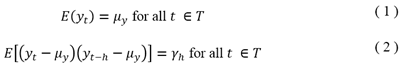Equation (1) simply implies that the expected values of the y process must have a constant mean, so the stationary process must fluctuate around a constant mean defined in µ, no trends are available in the process. Equation
(2) is telling us that variances are time-invariant, so the term γ, doesn’t depend on t but just on the distance h.

In order to get a better notion of stationarity, we define that a stationary process follows the pattern in the next graph. Which was generated using random values over a constant mean of 0, and with a normal probability distribution. The time period sample was n=500 observations.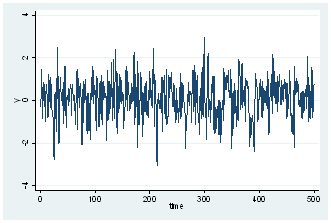The generated process fluctuates around a constant mean, and no tendency is present. How do we confirm if the series is normally distributed? Well, we can perform a histogram over the series. In Stata, the command is histogram y, norm where y is our variable.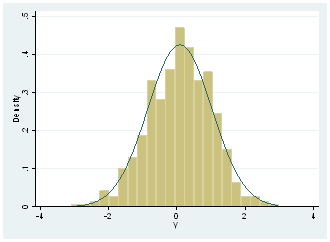The option of ,norm is given in Stata in order to present the actual normal distribution, so we can see that real distribution it’s not far from it. We can graphically affirm that series might present a normal distribution, but in order to confirm it, we need to do a formal test, so we perform Jarque-Bera test with the command sktest y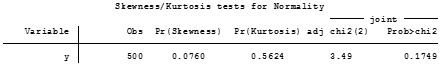The null hypothesis of the test is that normal distribution exists among the y variable And since p-value is bigger than a 5%significance level, we fail to reject null hypothesis and we can say that y variable is normally distributed.

Checking for unit roots also is useful when we’re trying to discover stationarity over a variable, so we perform first, the estimated ideal lag for the test, with varsoc y which will tell us what appropriated lag-length should be used in the ADF test.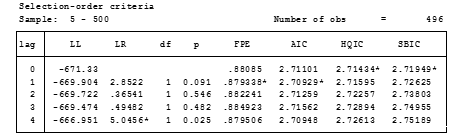Such results, indicate that ADF test over y variable must be done with one lag according to FPE, AIC, while HQIC and SBIC indicate 0 lags. It is the decision of the investigator to select the right information criteria (mostly it is selected when all error criteria are in a specific lag). However, we have a draw of FPE and AIC vs HQIC and SBIC. We will discard FPE since according to Liew (2004) this one is more suitable for samples lower than 120 observations, and thus we will select 0 lag for the test considering our sample size of 500 observations.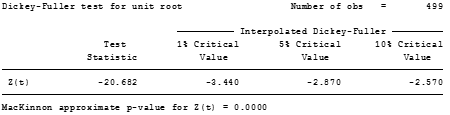Null hypothesis is the existence of unit roots in the variable, so we can strongly reject this and accept that no-unit roots are present. Sometimes this test is used to define stationarity of a respective process, but we need to take in consideration that stationarity involves constant means and normal distributions. We can say for now, that y variable is stationary.

At this point, one could argue Why we need the notion of stationarity over the residuals? This is because stationarity ensures that no spurious regressions are estimated. Now let’s assume we have a model which
follows an I (0) stationary model.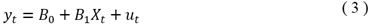And that I (0) variables are y and x, common intuition will tell us that u will be also stationary, but we need to ensure this. Proceeding with our Monte Carlo approaches, we generated the x series with a constant mean which has a normal distribution and that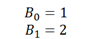with u ~ (0,1) as the Data Generating Process of y expressed in equation (3). Basically u has a mean of 0, and variance of 1. Regressing y on x we got the next result.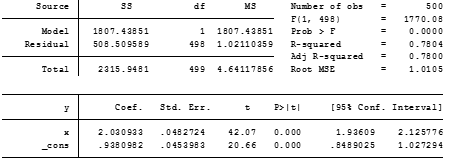We can see that coefficients B_0 and B_1 are approximated 1 and 2 respectively, so it’s almost close to the data generating process and both estimators are statistically significant at 1%. Let’s look at the residuals of the estimated model a little bit closer, we start by predicting the residuals using the command predict u, residuals in order to get the predicted values. Then we perform some of the tests we did before.

Graphic of the residuals with tsline u presentsthe next result, which looks like a stationary process.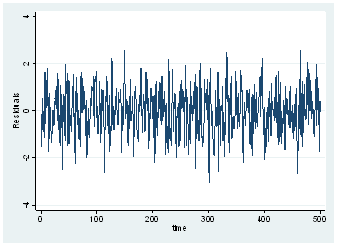A histogram over the residuals, will show the pattern
of normal distribution.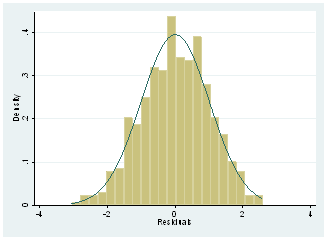And as well, the normality test will confirm this result.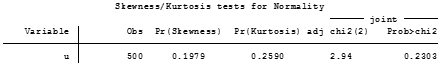Now we need to test that the residuals don’t follow a unit root pattern, a consideration here must be done first before we use ADF test, and is that critical values of the test are not applicable to the residuals. Thus, we cannot fully rely on this test.

In Stata we can recur to the Engle-Granger distribution test of the residuals, to whether accept or reject the idea that residuals are stationary. So, we type egranger y x which provides an accurate estimate of the critical values to evaluate the residuals.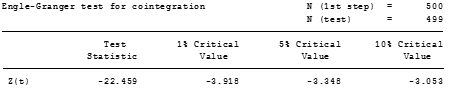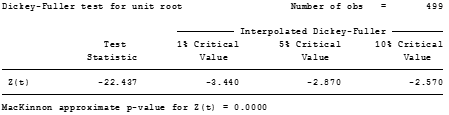As tests evidence, Test statistic is pretty close between ADF test and Engle & Granger test but the critical values are way different. Furthermore, we should rely on the results of the Engle & Granger test. Since Test statistic is bigger than 5% critical value, we can reject the null hypothesis that x and y are not cointegrated, and we can affirm that both variables present over this estimation a long run path of equilibrium. From another view, implies that the residuals are stationary and our regression is not spurious.

This basic idea can be extended with I (1) variables, in order to test whether it exists a long run path and if the regression model in (3) turns to be super consistent. Then long-run approximations with error correction forms can be done for this model where all variables are I (1).

This idea of testing residuals in stationary models is not a formal test used in the literature, however, it can reconfirm that with I (0) models that the regression will not be spurious. And it can also help to contrast long-run relationships.

Note: The package egranger must be installed first ssc install egranger, replace should do the trick. This package parts from the regression model to be estimated, however, it has the failure it cannot be computed with time operators. So, generating first differences or lagged values must be done in separate variables.

Bibliography

Liew, V. (2004). “Which Lag Length Selection Criteria Should We Employ?”. Journal of Economics Bulletin, 1-9. Recuperated from: